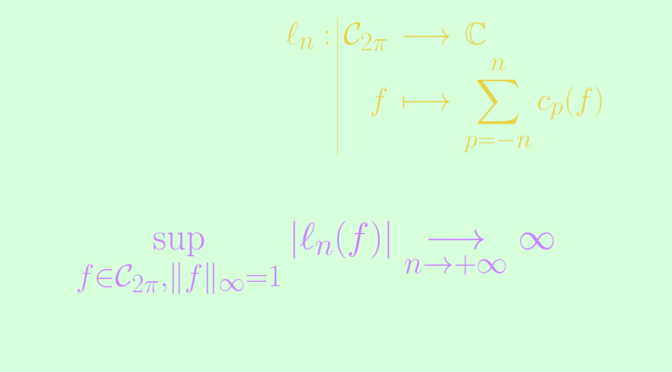# Existence of a continuous function with divergent Fourier series

In that article, I provided an example of a continuous function with divergent Fourier series. We prove here the existence of such a function using Banach-Steinhaus theorem, also called uniform boundedness principle.

Theorem (Uniform Boundedness Theorem) Let $$(X, \Vert \cdot \Vert_X)$$ be a Banach space and $$(Y, \Vert \cdot \Vert_Y)$$ be a normed vector space. Suppose that $$F$$ is a set of continuous linear operators from $$X$$ to $$Y$$. If for all $$x \in X$$ one has $\sup\limits_{T \in F} \Vert T(x) \Vert_Y \lt \infty$ then $\sup\limits_{T \in F, \ \Vert x \Vert = 1} \Vert T(x) \Vert_Y \lt \infty$

Let’s take for $$X$$ the vector space $$\mathcal C_{2 \pi}$$ of continuous functions from $$\mathbb R$$ to $$\mathbb C$$ which are periodic with period $$2 \pi$$ endowed with the norm $$\Vert f \Vert_\infty = \sup\limits_{- \pi \le t \le \pi} \vert f(t) \vert$$. $$(\mathcal C_{2 \pi}, \Vert \cdot \Vert_\infty)$$ is a Banach space. For the vector space $$Y$$, we take the complex numbers $$\mathbb C$$ endowed with the modulus.

For $$n \in \mathbb N$$, the map $\begin{array}{l|rcl} \ell_n : & \mathcal C_{2 \pi} & \longrightarrow & \mathbb C \\ & f & \longmapsto & \displaystyle \sum_{p=-n}^n c_p(f) \end{array}$ is a linear operator, where for $$p \in \mathbb Z$$, $$c_p(f)$$ denotes the complex Fourier coefficient $c_p(f) = \frac{1}{2 \pi} \int_{- \pi}^{\pi} f(t) e^{-i p t} \ dt$

We now prove that
\begin{align*}
\Lambda_n &= \sup\limits_{f \in \mathcal C_{2 \pi}, \Vert f \Vert_\infty=1} \vert \ell_n(f) \vert\\
&= \frac{1}{2 \pi} \int_{- \pi}^{\pi} \left\vert \frac{\sin (2n+1)\frac{t}{2}}{\sin \frac{t}{2}} \right\vert \ dt = \frac{1}{2 \pi} \int_{- \pi}^{\pi} \left\vert h_n(t) \right\vert \ dt,
\end{align*} where one can notice that the function $\begin{array}{l|rcll} h_n : & [- \pi, \pi] & \longrightarrow & \mathbb C \\ & t & \longmapsto & \frac{\sin (2n+1)\frac{t}{2}}{\sin \frac{t}{2}} &\text{for } t \neq 0\\ & 0 & \longmapsto & 2n+1 \end{array}$ is continuous.

Using the trigonometric equality $\displaystyle \sum_{p=-n}^n e^{-i p t} = \frac{\sin (2n+1)\frac{t}{2}}{\sin \frac{t}{2}}$ we get for $$f \in \mathcal C_{2 \pi}$$
\begin{align*}
\ell_n(f) &= \displaystyle \sum_{p=-n}^n c_p(f) = \frac{1}{2 \pi} \displaystyle \sum_{p=-n}^n \int_{- \pi}^{\pi} f(t) e^{-i p t} \ dt\\
&= \frac{1}{2 \pi} \int_{- \pi}^{\pi} \displaystyle f(t) \left(\sum_{p=-n}^n e^{-i p t} \right) \ dt\\
&= \frac{1}{2 \pi} \int_{- \pi}^{\pi} f(t) \frac{\sin (2n+1)\frac{t}{2}}{\sin \frac{t}{2}} \ dt = \frac{1}{2 \pi} \int_{- \pi}^{\pi} f(t) h_n(t) \ dt
\end{align*} hence the inequality $\Lambda_n \le \frac{1}{2 \pi} \int_{- \pi}^{\pi} \left\vert \frac{\sin (2n+1)\frac{t}{2}}{\sin \frac{t}{2}} \right\vert \ dt.$ For $$q \in \mathbb N$$ let’s note for $$t \in [-\pi,\pi]$$, $$\displaystyle f_q(t) = \frac{h_n(t)}{\vert h_n(t) \vert + \frac{1}{q}}$$. One can check that $$h_q \in \mathcal C_{2 \pi}$$. Then denoting $$\delta_q = \left\vert \ell_n(f_q) – \frac{1}{2 \pi} \int_{- \pi}^{\pi} \vert h_n(t) \vert \ dt \right\vert$$, we obtain
\begin{align*}
\delta_q & \le \frac{1}{2 \pi} \int_{- \pi}^{\pi} \left\vert \frac{h_n^2(t) – \vert h_n^2(t) \vert -\frac{1}{q}\vert h_n(t) \vert}{\vert h_n(t) \vert + \frac{1}{q}} \right\vert \ dt\\
& \le \frac{1}{2 q\pi} \int_{- \pi}^{\pi} \frac{\vert h_n(t) \vert}{\vert h_n(t) \vert + \frac{1}{q}} \ dt \le \frac{1}{q}
\end{align*} because $$h_n$$ is a real function. As $$\lim\limits_{q \to \infty} \Vert f_q \Vert = 1$$, following limits hold $\lim\limits_{q \to \infty} \ell_n(f_q/\Vert f_q \Vert) = \lim\limits_{q \to \infty} \ell_n(f_q) = \frac{1}{2 \pi} \int_{- \pi}^{\pi} \left\vert h_n(t) \right\vert \ dt$ which concludes our proof that $\Lambda_n = \sup\limits_{f \in \mathcal C_{2 \pi}, \Vert f \Vert_\infty=1} \vert \ell_n(f) \vert = \frac{1}{2 \pi} \int_{- \pi}^{\pi} \left\vert h_n(t) \right\vert \ dt$

Now for all $$t \in \mathbb R$$ $$\vert \sin t \vert \le \vert t \vert$$ and therefore
\begin{align*}
\Lambda_n & \ge \frac{1}{2 \pi} \int_{- \pi}^{\pi} \left\vert \frac{\sin (2n+1)\frac{t}{2}}{\frac{t}{2}} \right\vert \ dt = \frac{2}{\pi} \int_0^{\pi} \left\vert \frac{\sin (2n+1)\frac{t}{2}}{t} \right\vert \ dt\\
& \ge \frac{2}{\pi} \int_0^{(2n+1)\frac{\pi}{2}} \frac{\vert \sin u \vert}{u} \ du
\end{align*}

As it is known that $$\lim\limits_{x \to \infty} \int_0^x \frac{\vert \sin u \vert}{u} \ du = \infty$$ we get $\lim\limits_{n \to \infty} \Lambda_n = \infty$ This is were Banach-Steinhaus theorem comes into play. The set of linear continuous operators $$F=\{\ell_n \ ; \ n \in \mathbb N\}$$ is such that $\sup\limits_{\ell \in F, \ \Vert f \Vert_\infty = 1} \vert \ell(f) \vert = \infty.$ Therefore it exists $$f_0 \in \mathcal C_{2 \pi}$$ such that $$\sup\limits_{n \in \mathbb N} \vert \ell_n(f_0) \vert = \infty$$. It implies that the Fourier series of $$f_0$$ diverges at $$0$$ as if it was converging the sequence $$(\ell_n(f_0))$$ would be bounded.